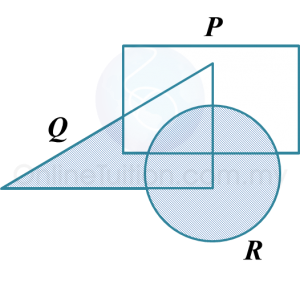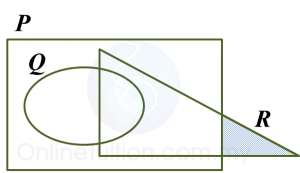# 4.5.4 Operations on Sets, SPM Paper 2 (Long Questions)

Question 7:
The Venn diagrams in the answer space shows sets P, Q and R such that the universal set, $\xi =P\cup Q\cup R$ .
(a) P Q,
(b) ${P}^{‘}\cap Q\cup R$Solution:
(a)
P Q(b)
${P}^{‘}\cap Q\cup R$Question 8:
The Venn diagrams in the answer space shows sets P, Q and R such that the universal set, $\xi =P\cup Q\cup R$ .
(a) ${Q}^{‘}\cap R$
(b) $\left(Q\cup R\right)\cap {P}^{‘}$Solution:
(a)
${Q}^{‘}\cap R$(b)
$\left(Q\cup R\right)\cap {P}^{‘}$